## Equations of Lines

Equations involving one or two variables can be graphed on any x− y coordinate plane. In general, the following principles are true:

• If a point lies on the graph of an equation, then its coordinates make the equation a true statement.
• If the coordinates of a point make an equation a true statement, then the point lies on the graph of the equation.

linear equation is any equation whose graph is a line. All linear equations can be written in the form Ax + By = C where A, B, and C are real numbers and A and B are not both zero. The following examples are linear equations and their respective A, B, and C values.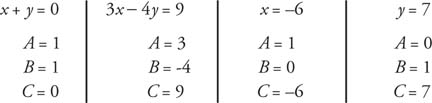This form for equations of lines is known as the standard form for the equation of a line.

The ‐intercept of a graph is the point where the graph intersects the x‐axis. It always has a y‐coordinate of zero. A horizontal line that is not the x‐axis has no x‐intercept .

The ‐intercept of a graph is the point where the graph intersects the y‐axis. It always has an x‐coordinate of zero. A vertical line that is not the y‐axis has no y‐intercept .

One way to graph a linear equation is to find solutions by giving a value to one variable and solving the resulting equation for the other variable. A minimum of two points is necessary to graph a linear equation.

Example 1: Draw the graph of 2 x + 3 y = 12 by finding the x‐intercept and the y‐intercept .

The x‐intercept has a y‐coordinate of zero. Substituting zero for y, the resulting equation is 2 x+ 3(0) = 12. Now solving for x,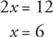The x‐intercept is at (6, 0), or the x‐intercept value is 6.

The y‐intercept has an x‐coordinate of zero. Substituting zero for x, the resulting equation is 2(0) + 3 y = 12. Now solving for y,The y‐intercept is at (0, 4), or the y‐intercept value is 4.

The line can now be graphed by graphing these two points and then drawing the line they determine (Figure 1).Figure 1 Drawing the graph of a linear equation after finding the x‐intercept and the y‐intercept .

Example 2: Draw the graph of x = 2.

x = 2 is a vertical line whose x‐coordinate is always 2 (Figure 2.Figure 2 The graph of a vertical line.

Example 3: Draw the graph of y = −1.

y = −1 is a horizontal line whose y‐coordinate is always −1. See Figure 2.Figure 3

Suppose that A is a particular point called ( x 1y 1) and B is any point called ( x, y). Then the slope of the line through A and B is represented by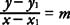Applying the Cross‐Products Propertyy − y 1 = m ( x − x 1). This is the point‐slope form of a nonvertical line.

Theorem107: The point‐slope form of a line passing through ( x 1y 1) and having slope m is y − y 1 = m ( x − x 1).

Example 4: Find the equation of a line containing the points (−3,4) and (7,2) and write the equation in a. a. (a) point‐slope form and (b) standard form.

a. (a) For the point‐slope form, first find the slope, m.Now choose either original point—say, (−3, 4).

So,b. (b) Begin with the point‐slope form and clear it of fractions by multiplying both sides by the least common denominator.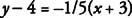Multiply both sides by 5.Get x and y on one side and the constants on the other side by adding x to both sides and adding 20 to both sides.A nonvertical line written in standard form is Ax + By = C with B ≠ 0. If this equation is solved for y, it becomes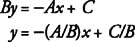Let b denote the y‐intercept of a line. The point‐slope form of the equation of the line passing through (0, b) with slope m is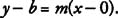Adding b to both sides of the equation yields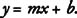This is known as the slope‐intercept form of the equation of a nonvertical line. Note that, in order to obtain the slope‐intercept form, a nonvertical line written in standard form Ax + By = C with B ≠ 0 can be solved algebraically for y.

Theorem 108: The slope‐intercept form of a nonvertical line with slope m and y‐intercept value b is y = mx + b.

Example 5: Find the slope and y‐intercept value of the line with equation 3 x − 4 y = 20.Therefore, the slope of the line is 3/4 and the y‐intercept value is −5.

Example 6: Line l 1 has equation 2 x + 5 y = 10. Line l 2 has equation 4 x + 10 y = 30. Line l 3 has equation 15 x − 6 y = 12. Which lines, if any, are parallel?

Put each equation into slope‐intercept form and determine the slope of each line.

• l 1• l 2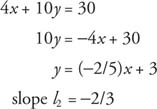• l 3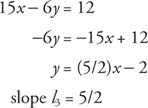Slope l 1 = slope l 2, therefore; l 1 // l 2 by Theorem 104.

Because (slope l 1)(slope l 3) = −1 and (slope l 2)(slope l 3) = −1, l 1 ⊥ l 3 and l 2 ⊥ l 3 by Theorem 106.